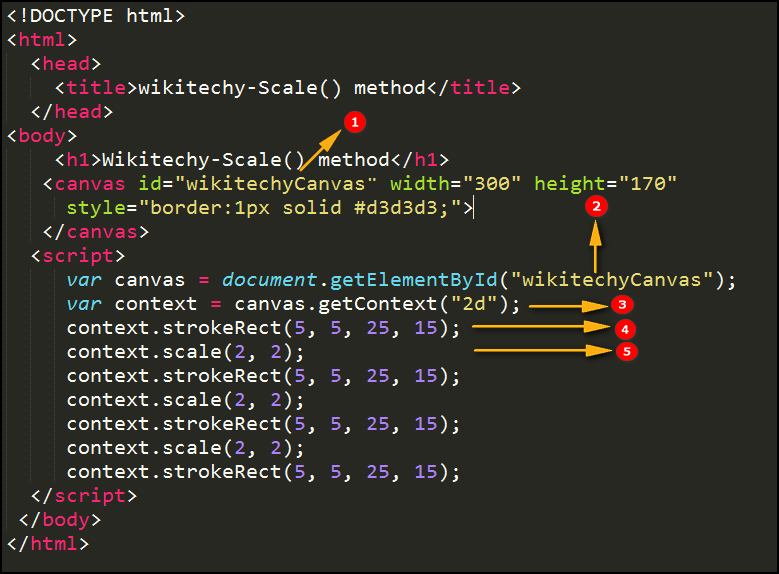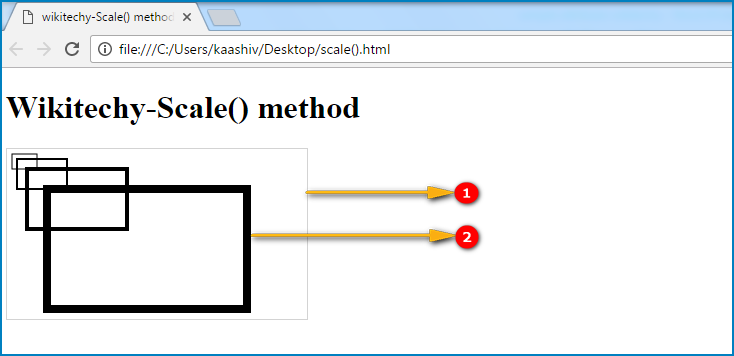# html tutorial - scale() Method in HTML5 Canvas - html5 - html code - html formLearn html - html tutorial - Scale method in html5 canvas - html examples - html programs

• The scale() is the Method of HTML canvas.
• The scale() method is used to set the size of the diagram(bigger or smaller).

## Parameter values for scale() Method in HTML5 Canvas:

Parameter Description
scalewidth Scales the width of the current drawing (1=100%, 0.5=50%, 2=200%, etc.)
scaleheight Scales the height of the current drawing (1=100%, 0.5=50%, 2=200%, etc.)

## Code Explanation for scale() Method in HTML5 Canvas:1. ”wikitechyCanvas” is used to declare the id value of the <canvas> tag.
2. The getElementById(); method is used to get the element with the specific id “wikitechyCanvas”.
3. The Canvas.getContext(“2d”)) method returns an object that provides methods and properties for drawing a two-dimension diagram on the canvas.
4. The strokeRect() method is used to begins the path for a line.
5. The scale() method is used to scales the current drawing size as (2,2).

## Output for scale() Method in HTML5 Canvas:1. The output shows that a canvas rectangle with gray color border.
2. It shows scale of the rectangle by using the scale() method.

## Browser Support for scale() Method in HTML5 Canvas:Yes 9.0 Yes Yes Yes

## Tips and Notes

• If you scale a drawing, all future drawings will also be scaled.
• The positioning will also be scaled. If you scale (3,3); drawings will be positioned thrice as far from the left and top of the canvas as you specify